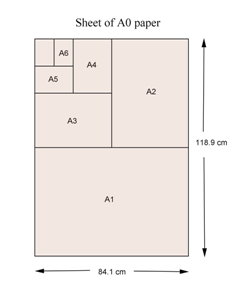All A grade sheets of paper are rectangles whose sides are in the ratio $\sqrt{2}:1.$

The area of an A0 sheet is 1 square metre.

Folding an 'Ax' sheet of paper in half produces an 'A(x+1)' sheet.

Therefore, the area of an 'Ax' sheet of paper is $(\frac{1}{2})^x$ square metres.

The actual dimensions of A4 are 297 mm x 210 mm. We can compute the dimensions as $\left[\frac{250\sqrt2}{\sqrt(\sqrt2)}\right]$ mm and $\left[\frac{250}{\sqrt(\sqrt2)}\right]$ mm, which are 297.3017 ... mm and 210.2241 ... mm respectively. Very, very close.

The ratio of sides is an irrational number, just as for a golden rectangle.

(none) (none) (none)
(none) (none)

## You are here

A4Paper
IrrationalNumber
(none) (none) Integer
RationalNumber
RealNumber
SquareRoot
TranscendentalNumber